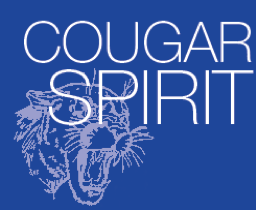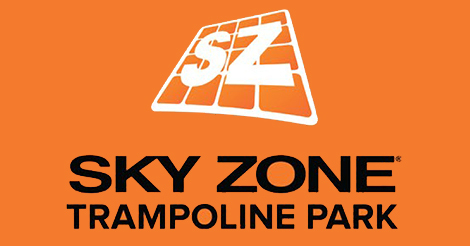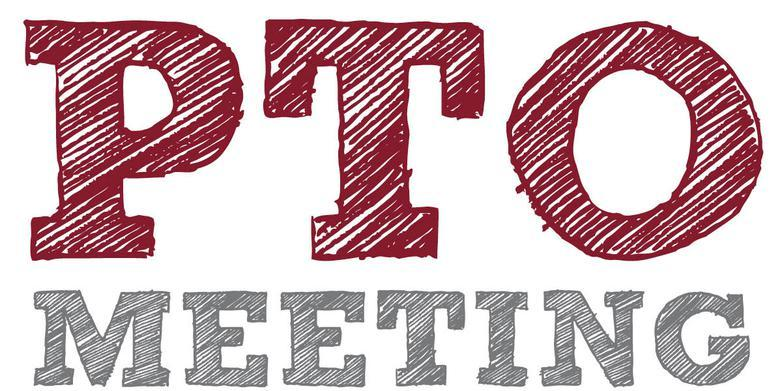/* styles */ Hi DC Community!

# The Desert Cove eNews is sent on a MONTHLY basis.

If you do not wish to receive the Desert Cove PTO eNews,
please click 'Unsubscribe' at the bottom of this emailMAY 2019

▪ Apr 29-May 3: DC Spirit Week & Food Drive by StuCo
▪ Apr 29-May 3: BOGO Book Fair (VOLUNTEERS NEEDED)
▪ May 1: Principal's Day
▪ May 3: DC Spirit Night @ Sky Zone
▪ May 6-10: Teacher Appreciation Week
▪ May 8: School Nurse's Day
▪ May 14: PTO Meeting @ 6PM in Library
▪ May 15: PVUPC Meeting
▪ May 23: EARLY RELEASE & LAST DAY OF SCHOOL
 ▪ Apr 29-May 3: DC Spirit Week & Food Drive by StuCo
 ▪ Apr 29-May 3: BOGO Book Fair (VOLUNTEERS NEEDED)
 ▪ May 1: Principal's Day
 ▪ May 3: DC Spirit Night @ Sky Zone
 ▪ May 6-10: Teacher Appreciation Week
 ▪ May 8: School Nurse's Day
 ▪ May 14: PTO Meeting @ 6PM in Library
 ▪ May 15: PVUPC Meeting
 ▪ May 23: EARLY RELEASE & LAST DAY OF SCHOOL

 table div table+table+table+table+table+table div table{width:100%;padding:0}table div table+table+table+table+table+table div table img{width:96.23%;padding:0;float:none}table div table+table+table+table+table+table div table td{width:100%;padding:0 1.88% 18px}/* styles */table.module-6{width:99.06%;padding:0}table div table+table+table+table+table+table+table div table{width:99.06%;float:none;margin-left:auto;margin-right:auto;padding:0}table div table+table+table+table+table+table+table div table a{border:0 none;text-decoration:none}table div table+table+table+table+table+table+table div table img{width:100%!important;border:0 none;text-decoration:none}table div table+table+table+table+table+table+table div table td{width:100%;padding:0}/* styles */

# The Desert Cove PTO communicates information in many ways to our DC Families!Pick one or more that work best for your family!Click the image below for more details!

 table div table+table+table+table+table+table+table+table+table div table td,table.module-8{width:100%;padding:0}table div table+table+table+table+table+table+table+table+table div table{width:100%;float:none;margin-left:auto;margin-right:auto;padding:0}table div table+table+table+table+table+table+table+table+table div table a{border:0 none;text-decoration:none}table div table+table+table+table+table+table+table+table+table div table img{width:100%!important;border:0 none;text-decoration:none}/* styles */
 table div table+table+table+table+table+table+table+table+table+table div table{width:100%;padding:0}table div table+table+table+table+table+table+table+table+table+table div table img{width:96.23%;padding:0;float:none}table div table+table+table+table+table+table+table+table+table+table div table td{width:100%;padding:0 1.88% 18px}/* styles */## APR 29 - MAY 3: Scholastic BOGO Book Fair## Mon. 4/29 - Set up Day

▪ We need Volunteers to help set up the Book Fair
 ▪ We need Volunteers to help set up the Book Fair

## Tue. 4/30 - Preview Day

▪ We need Volunteers to help students write wish lists.
 ▪ We need Volunteers to help students write wish lists.

## Wed. 5/1 - Fri. 5/3 - Book Fair is Open for Business!

▪ We need Volunteers to cashier and restock bookshelves
 ▪ We need Volunteers to cashier and restock bookshelves
 table div table+table+table+table+table+table+table+table+table+table+table+table+table+table div table{width:100%;padding:0}table div table+table+table+table+table+table+table+table+table+table+table+table+table+table div table img{width:96.23%;padding:0;float:none}table div table+table+table+table+table+table+table+table+table+table+table+table+table+table div table td{width:100%;padding:0 1.88% 18px}/* styles */## APR 29 - MAY 3: DC Spirit Week & Food DriveBrought to you by Student CouncilMon. 4/29 - Pajama Day
Tue. 4/30 - Twin Day
Wed. 5/1 - Sports Day
Thu. 5/2 - Tourist Day
Fri. 5/3 - Look Towards the Future

We are also doing a food drive to benefit Paradise Valley Food Bank. The class that brings in the most items wins a pizza party!

Please bring in non-perishable items during the week of April 29th-May 3rd.

 table div table+table+table+table+table+table+table+table+table+table+table+table+table+table+table+table+table div table{width:100%;padding:0}table div table+table+table+table+table+table+table+table+table+table+table+table+table+table+table+table+table div table img{width:96.23%;padding:0;float:none}table div table+table+table+table+table+table+table+table+table+table+table+table+table+table+table+table+table div table td{width:100%;padding:0 1.88% 18px}/* styles */## MAY 3: DC Spirit Night @ Sky Zone4pm-8pmtable div table+table+table+table+table+table+table+table+table+table+table+table+table+table+table+table+table+table+table+table+table div table{width:100%;padding:0}table div table+table+table+table+table+table+table+table+table+table+table+table+table+table+table+table+table+table+table+table+table div table img{width:96.23%;padding:0;float:none}table div table+table+table+table+table+table+table+table+table+table+table+table+table+table+table+table+table+table+table+table+table div table td{width:100%;padding:0 1.88% 18px}/* styles */## MAY 1 - 10: Teacher & Staff Appreciation Days## May 8: School Nurse's Day

May is the month to show appreciation for all Desert Cove Teachers & Staff! Click Here for some Ideas for gifts that the kids can make.

 table div table+table+table+table+table+table+table+table+table+table+table+table+table+table+table+table+table+table+table+table+table+table+table+table div table{width:100%;padding:0}table div table+table+table+table+table+table+table+table+table+table+table+table+table+table+table+table+table+table+table+table+table+table+table+table div table img{width:96.23%;padding:0;float:none}table div table+table+table+table+table+table+table+table+table+table+table+table+table+table+table+table+table+table+table+table+table+table+table+table div table td{width:100%;padding:0 1.88% 18px}/* styles */## MAY 14: PTO MEETING# FREE Pizza & Childcare provided!

## If you are interested in a Board or Chair position, please come to this meeting!

Can't make the meeting? No worries, Meeting Minutes are posted on our website:

www.desertcovepto.org/meetings

 table div table+table+table+table+table+table+table+table+table+table+table+table+table+table+table+table+table+table+table+table+table+table+table+table+table+table+table+table div table{width:100%;padding:0}table div table+table+table+table+table+table+table+table+table+table+table+table+table+table+table+table+table+table+table+table+table+table+table+table+table+table+table+table div table img{width:96.23%;padding:0;float:none}table div table+table+table+table+table+table+table+table+table+table+table+table+table+table+table+table+table+table+table+table+table+table+table+table+table+table+table+table div table td{width:100%;padding:0 1.88% 18px}/* styles */## A big THANK YOU to all the DC Families & Friends that came out to our Free Family Movie Night! It was a BLAST!

And another big THANK YOU again to our Sponsors:

 table.module-30{width:99.81%;padding:0}table div table+table+table+table+table+table+table+table+table+table+table+table+table+table+table+table+table+table+table+table+table+table+table+table+table+table+table+table+table+table+table div table{width:99.81%;float:none;margin-left:auto;margin-right:auto;padding:0}table div table+table+table+table+table+table+table+table+table+table+table+table+table+table+table+table+table+table+table+table+table+table+table+table+table+table+table+table+table+table+table div table a{border:0 none;text-decoration:none}table div table+table+table+table+table+table+table+table+table+table+table+table+table+table+table+table+table+table+table+table+table+table+table+table+table+table+table+table+table+table+table div table img{width:100%!important;border:0 none;text-decoration:none}table div table+table+table+table+table+table+table+table+table+table+table+table+table+table+table+table+table+table+table+table+table+table+table+table+table+table+table+table+table+table+table div table td{width:100%;padding:0}/* styles */
 table div table+table+table+table+table+table+table+table+table+table+table+table+table+table+table+table+table+table+table+table+table+table+table+table+table+table+table+table+table+table+table+table div table{width:100%;padding:0}table div table+table+table+table+table+table+table+table+table+table+table+table+table+table+table+table+table+table+table+table+table+table+table+table+table+table+table+table+table+table+table+table div table img{width:96.23%;padding:0;float:none}table div table+table+table+table+table+table+table+table+table+table+table+table+table+table+table+table+table+table+table+table+table+table+table+table+table+table+table+table+table+table+table+table div table td{width:100%;padding:0 1.88% 18px}/* styles */We have created a page on the PTO website where you can access the following communications:

Desert Cove PTO eNews archives
Desert Cove Principal News
PV School District eNews
United Parent Council eNews